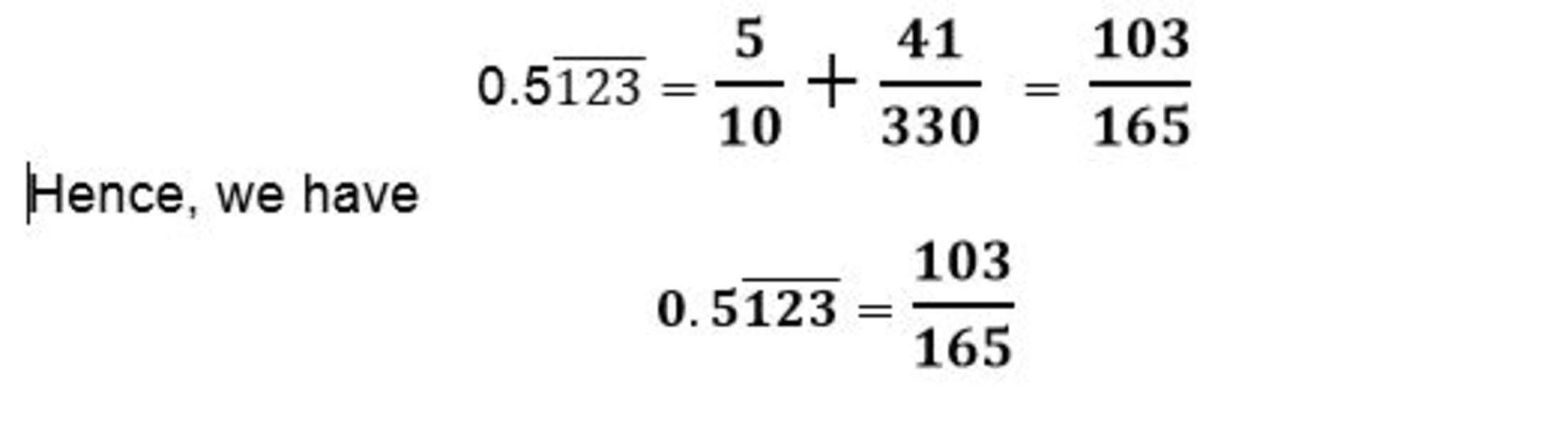## Step 1: Count the number of recurring digits

For example, if we want to change 0.567567 to a fraction, there are three recurring digits: 5, 6 and 7.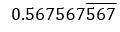## Step 2: Convert to a fraction

We write the first set of the recurring digits as the numerator and the denominator with a series of 9s, depending on the count from Step 1. Since we counted three and we have 567 as the numerator, the denominator would have three 9s.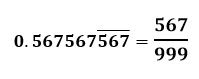## Step 3: Simplify the fraction

Cancel common factors to simplify the fraction.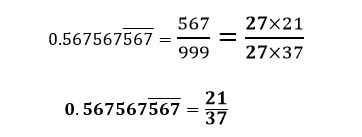## Examples of how to work out recurring decimals

Q) Express the following recurring decimals to fractions.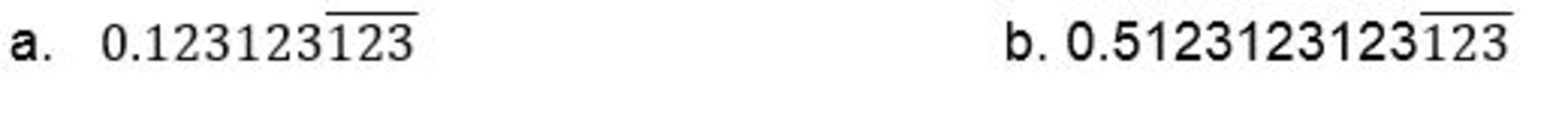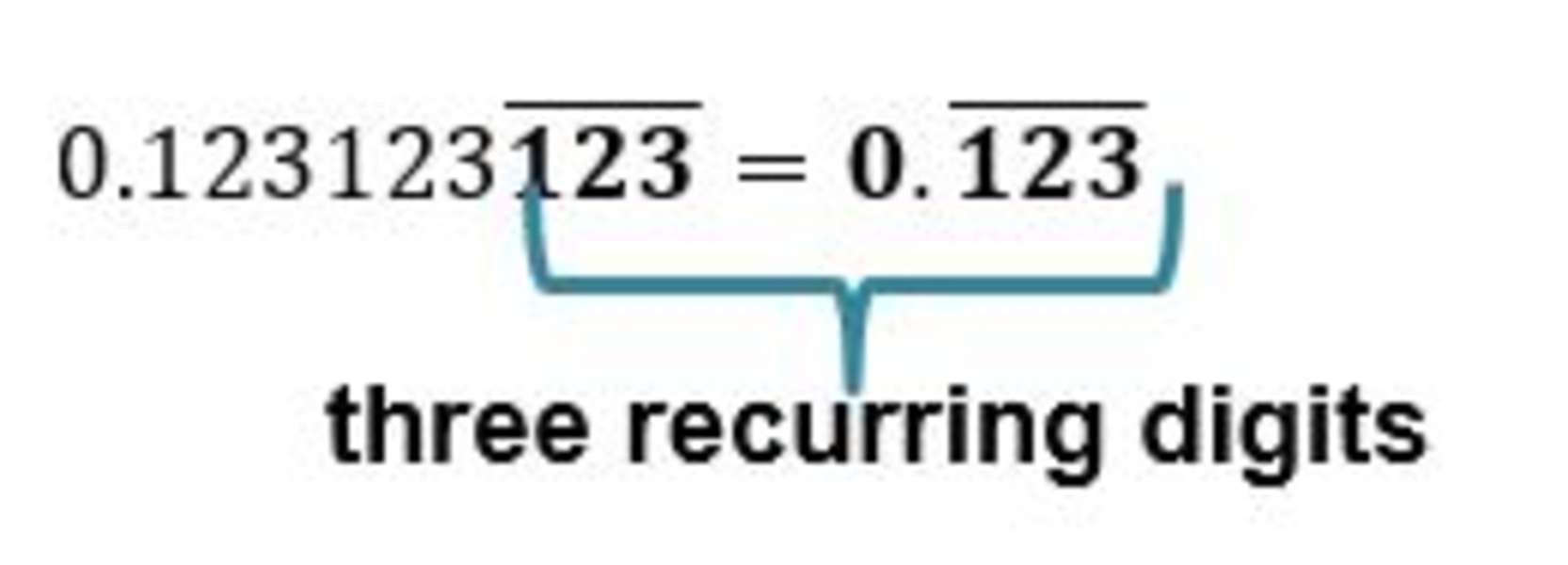a. To change 0.123123 to a fraction, we first count the number of digits that are recurring.

We would have three 9s on the denominator and 123 as the numerator.

0.(123) ̅=123/999

Look for common factors shared by the numerator and denominator to simplify the fraction.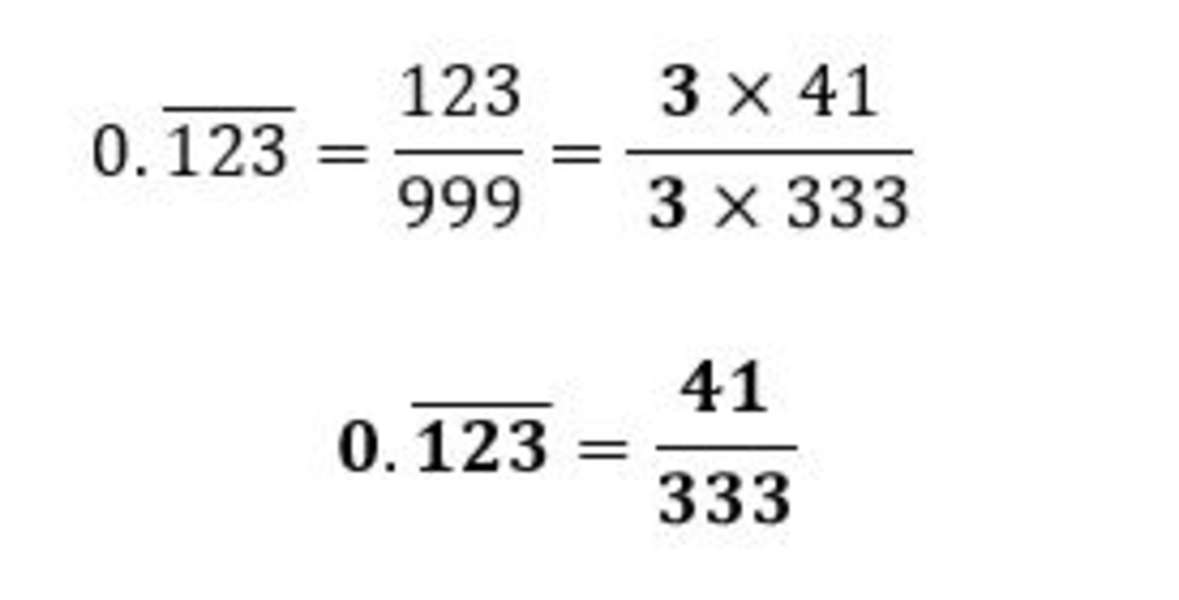b. Now to change 0.5123123123, we first separate the recurring and non-recurring digits.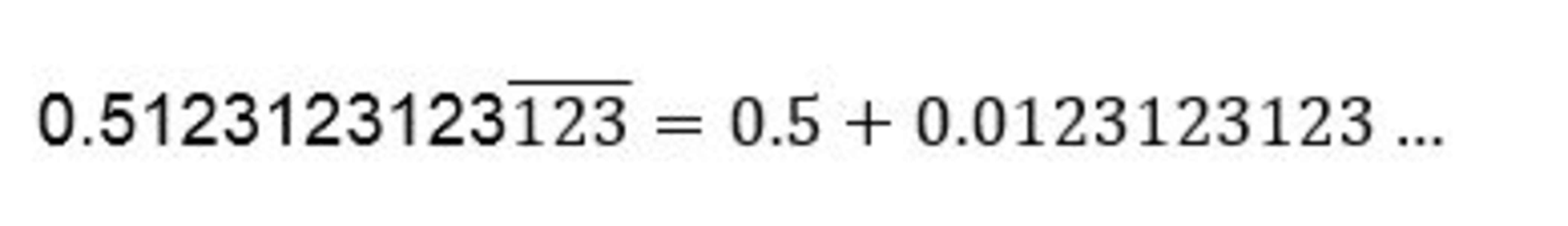Express 0.5 as a fraction: .5= 5/10.

To express 0.0123123 as a fraction, we can use the value of 123 from part a. So, we have 123 ̅= 123/99=41/33.

Since there’s only 1 zero before the recurring digits, we multiply 41/33 by 1/10.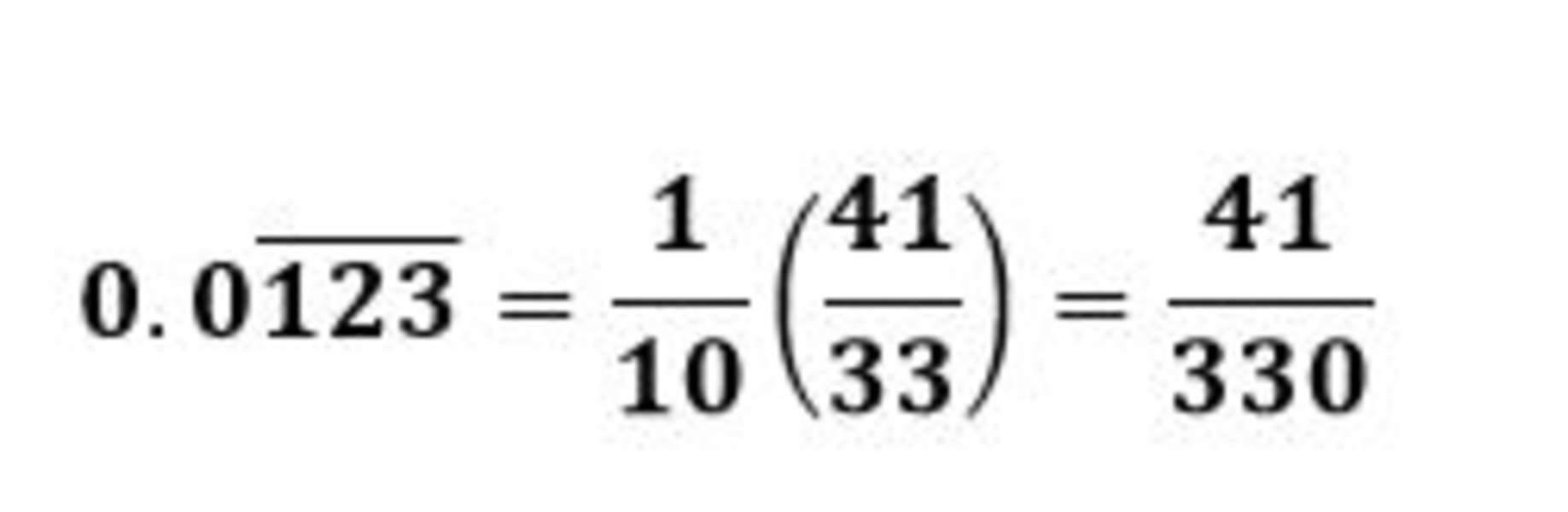Now, to find the value of 0.5(123) ̅, we combine the values of the decimals.